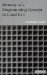# Exercises7.1 We discussed static two-dimensional arrays (and their row-major storing) as well as implementation of two-dimensional dynamic arrays. Static and dynamic arrays both use the same "syntax" for access (e.g., x ); how is this possible given the totally different storage methods ?

7.2 Write a simple C program in which a two-dimensional dynamic array is used. Now add a function that adds a new row to the array. How complicated was it?

7.3 Take the program from Exercise 7.2 and add a function that adds a new column to the array. How complicated was this in comparison to Exercise 7.2?

7.4 Implement a dynamic array of strings in the form of a dynamic array of pointers to strings. Use an implementation that is similar to a two-dimensional character array, where each string is stored in a row of different size .

7.5 In C, use the row-major approach to implement an integer dynamic two-dimensional pseudo-array that is always stored in a contiguous segment of memory. For access you must use two functions, Get(i,j) (to fetch a value) and Set(i,j,value) ; it is because you need these two access functions that we use the term pseudo -array. In C++, implement this as a class that allows the proper indexing x[i][j] .Memory as a Programming Concept in C and C++
ISBN: 0521520436
EAN: 2147483647
Year: 2003
Pages: 64

Similar book on Amazon

flylib.com © 2008-2017.
If you may any questions please contact us: flylib@qtcs.net